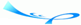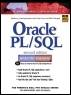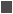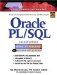# Lab 5.3 Nested IF Statements

 Team-FlyOracle® PL/SQL® Interactive Workbook, Second EditionBy Benjamin Rosenzweig, Elena Silvestrova Table of Contents Chapter 5.  Conditional Control: IF Statements

Lab Objectives

After this Lab, you will be able to:

• Use Nested IF Statements

You have encountered different types of conditional controls: IF-THEN statement, IF-THEN-ELSE statement, and ELSIF statement. These types of conditional controls can be nested inside of anotherfor example, an IF statement can be nested inside an ELSIF and vice versa. Consider the following:FOR EXAMPLE

` DECLARE     v_num1 NUMBER := &sv_num1;     v_num2 NUMBER := &sv_num2;     v_total NUMBER;  BEGIN     IF v_num1 > v_num2 THEN        DBMS_OUTPUT.PUT_LINE ('IF part of the outer IF');        v_total := v_num1 - v_num2;     ELSE        DBMS_OUTPUT.PUT_LINE ('ELSE part of the outer IF');        v_total := v_num1 + v_num2;        IF v_total < 0 THEN           DBMS_OUTPUT.PUT_LINE ('Inner IF');           v_total := v_total * (-1);        END IF;     END IF;     DBMS_OUTPUT.PUT_LINE ('v_total = '||v_total);  END; `

The IF-THEN-ELSE statement is called an outer IF statement because it encompasses the IF-THEN statement (shown in bold letters). The IF-THEN statement is called an inner IF statement because it is enclosed by the body of the IF-THEN-ELSE statement.

Assume that the value for v_num1 and v_num2 are -4 and 3 respectively. First, the condition

` v_num1 > v_num2 `

of the outer IF statement is evaluated. Since -4 is not greater than 3, the ELSE part of the outer IF statement is executed. As a result, the message

` ELSE part of the outer IF `

is displayed, and the value of v_total is calculated. Next, the condition

` v_total < 0 `

of the inner IF statement is evaluated. Since that value of v_total is equal -l, the condition yields TRUE, and message

` Inner IF `

is displayed. Next, the value of v_total is calculated again. This logic is demonstrated by the output produced by the example:

` Enter value for sv_num1: -4  old   2:    v_num1  NUMBER := &sv_num1;  new   2:    v_num1  NUMBER := -4;  Enter value for sv_num2: 3  old   3:    v_num2  NUMBER := &sv_num2;  new   3:    v_num2  NUMBER := 3;  ELSE part of the outer IF  Inner IF  v_total = 1  PL/SQL procedure successfully completed. `

#### Logical Operators

So far in this chapter, you have seen examples of different IF statements. All of these examples used test operators, such as >, <, and =, to test a condition. Logical operators can be used to evaluate a condition, as well. In addition, they allow a programmer to combine multiple conditions into a single condition if there is such a need.FOR EXAMPLE

` DECLARE     v_letter CHAR(1) := '&sv_letter';  BEGIN     IF (v_letter >= 'A' AND v_letter <= 'Z') OR        (v_letter >= 'a' AND v_letter <= 'z')     THEN        DBMS_OUTPUT.PUT_LINE ('This is a letter');     ELSE        DBMS_OUTPUT.PUT_LINE ('This is not a letter');        IF v_letter BETWEEN '0' and '9' THEN           DBMS_OUTPUT.PUT_LINE ('This is a number');        ELSE           DBMS_OUTPUT.PUT_LINE ('This is not a number');        END IF;     END IF;  END; `

In this example, the condition

` (v_letter >= 'A' AND v_letter <= 'Z') OR  (v_letter >= 'a' AND v_letter <= 'z') `

uses logical operators AND and OR. There are two conditions

` (v_letter >= 'A' AND v_letter <= 'Z') `

and

` (v_letter >= 'a' AND v_letter <= 'z') `

combined into one with the help of the OR operator. It is also important for you to realize the purpose of the parentheses. In this example, they are used to improve readability only, because the operator AND takes precedence over the operator OR.

When the symbol "?" is entered at runtime, this example produces the following output:

` Enter value for sv_letter: ?  old   2:    v_letter CHAR(1) := '&sv_letter';  new   2:    v_letter CHAR(1) := '?';  This is not a letter  This is not a number  PL/SQL procedure successfully completed. `

#### Lab 5.3 Exercises

##### 5.3.1 Use Nested IF Statements

In this exercise, you will use nested IF statements. This script will convert the value of a temperature from one system to another. If the temperature is supplied in Fahrenheit, it will be converted to Celsius, and vice versa.

Create the following PL/SQL script:

` -- ch05_4a.sql, version 1.0  SET SERVEROUTPUT ON  DECLARE     v_temp_in NUMBER := &sv_temp_in;     v_scale_in CHAR := '&sv_scale_in';     v_temp_out NUMBER;     v_scale_out CHAR;  BEGIN     IF v_scale_in != 'C' AND v_scale_in != 'F' THEN        DBMS_OUTPUT.PUT_LINE ('This is not a valid scale');     ELSE        IF v_scale_in = 'C' THEN           v_temp_out := ( (9 * v_temp_in) / 5 ) + 32;           v_scale_out := 'F';        ELSE           v_temp_out := ( (v_temp_in  32) * 5 ) / 9;           v_scale_out := 'C';        END IF;        DBMS_OUTPUT.PUT_LINE ('New scale is: '||           v_scale_out);        DBMS_OUTPUT.PUT_LINE ('New temperature is: '||           v_temp_out);     END IF;  END; `

Execute the script, and then answer the following questions:

 a) What output is printed on the screen if the value of 100 is entered for the temperature, and the letter "C" is entered for the scale? b) Try to run this script without providing a value for the temperature. What message will be displayed on the screen? Why? c) Try to run this script providing an invalid letter for the temperature scale, for example, letter "V." What message will be displayed on the screen? Why? d) Rewrite this script so that if an invalid letter is entered for the scale, v_temp_out is initialized to zero and v_scale_out is initialized to C.

#### Lab 5.3 Exercise Answers

This section gives you some suggested answers to the questions in Lab 5.3, with discussion related to how those answers resulted. The most important thing to realize is whether your answer works. You should figure out the implications of the answers here and what the effects are from any different answers you may come up with.

##### 5.3.1 Answers

 a) What output is printed on the screen if the value of 100 is entered for the temperature, and the letter "C" is entered for the scale? A1: Answer: Your output should look like the following:` Enter value for sv_temp_in: 100 old 2: v_temp_in NUMBER := &sv_temp_in; new 2: v_temp_in NUMBER := 100; Enter value for sv_scale_in: C old 3: v_scale_in CHAR := '&sv_scale_in'; new 3: v_scale_in CHAR := 'C'; New scale is: F New temperature is: 212 PL/SQL procedure successfully completed. `Once the values for v_temp_in and v_scale_in have been entered, the condition` v_scale_in != 'C' AND v_scale_in != 'F' `of the outer IF statement evaluates to FALSE, and control is passed to the ELSE part of the outer IF statement. Next, the condition` v_scale_in = 'C' `of the inner IF statement evaluates to TRUE, and the values of the variables v_temp_out and v_scale_out are calculated. Control is then passed back to the outer IF statement, and the new value for the temperature and the scale are displayed on the screen. b) Try to run this script without providing a value for the temperature. What message will be displayed on the screen? Why? A2: Answer: If the value for the temperature is not entered, the script will not compile at all.The compiler will try to assign a value to v_temp_in with the help of the substitution variable. Because the value for v_temp_in has not been entered, the assignment statement will fail, and the following error message will be displayed.` Enter value for sv_temp_in: old 2: v_temp_in NUMBER := &sv_temp_in; new 2: v_temp_in NUMBER := ; Enter value for sv_scale_in: C old 3: v_scale_in CHAR := '&sv_scale_in'; new 3: v_scale_in CHAR := 'C'; v_temp_in NUMBER := ; * ERROR at line 2: ORA-06550: line 2, column 27: PLS-00103: Encountered the symbol ";" when expecting one of the following: ( - + mod not null avg count current exists max min prior sql stddev sum variance cast The symbol "null" was substituted for ";" to continue. `You have probably noticed that even though the mistake seems small and insignificant, the error message is fairly long and confusing. c) Try to run this script providing an invalid letter for the temperature scale, for example, letter "V." What message will be displayed on the screen? Why? A3: Answer: If an invalid letter is entered for the scale, the message "This is not a valid scale" will be displayed on the screen.The condition of the outer IF statement will evaluate to TRUE. As a result, the inner IF statement will not be executed at all, and the message "This is not a valid scale" will be displayed on the screen.Assume that letter "V" was typed by mistake. This example will produce the following output:` Enter value for sv_temp_in: 45 old 2: v_temp_in NUMBER := &sv_temp_in; new 2: v_temp_in NUMBER := 45; Enter value for sv_scale_in: V old 3: v_scale_in CHAR := '&sv_scale_in'; new 3: v_scale_in CHAR := 'V'; This is not a valid scale PL/SQL procedure successfully completed. ` d) Rewrite this script so that if an invalid letter is entered for the scale, v_temp_out is initialized to zero and v_scale_out is initialized to C. A4: Answer: Your script should look similar to the following script. Changes are shown in bold letters. Notice that the two last DBMS_OUTPUT.PUT_LINE statements have been moved from the body of the outer IF statement.` -- ch05_4b.sql, version 2.0 DECLARE v_temp_in NUMBER := &sv_temp_in; v_scale_in CHAR := '&sv_scale_in'; v_temp_out NUMBER; v_scale_out CHAR; BEGIN IF v_scale_in != 'C' AND v_scale_in != 'F' THEN DBMS_OUTPUT.PUT_LINE ('This is not a valid scale'); v_temp_out := 0; v_scale_out := 'C'; ELSE IF v_scale_in = 'C' THEN v_temp_out := ( (9 * v_temp_in) / 5 ) + 32; v_scale_out := 'F'; ELSE v_temp_out := ( (v_temp_in - 32) * 5 ) / 9; v_scale_out := 'C'; END IF; END IF; DBMS_OUTPUT.PUT_LINE ('New scale is: '||v_scale_out); DBMS_OUTPUT.PUT_LINE ('New temperature is: '|| v_temp_out); END; `The preceding script produces the following output:` Enter value for sv_temp_in: 100 old 2: v_temp_in NUMBER := &sv_temp_in; new 2: v_temp_in NUMBER := 100; Enter value for sv_scale_in: V old 3: v_scale_in CHAR := '&sv_scale_in'; new 3: v_scale_in CHAR := 'V'; This is not a valid scale. New scale is: C New temperature is: 0 PL/SQL procedure successfully completed. `

#### Lab 5.3 Self-Review Questions

In order to test your progress, you should be able to answer the following questions.

Answers appear in Appendix A, Section 5.3.

 1) What types of IF statements can be nested one inside another? _____ IF-THEN statement can only be nested inside ELSIF statement._____ IF-THEN-ELSE statement cannot be nested at all._____ Any IF statement can be nested inside another IF statement. 2) How many IF statements can be nested one inside another? _____ One_____ Two_____ Any number 3) Only a single logical operator can be used with a condition of an IF statement. _____ True_____ False 4) When using nested IF statements, their conditions do not need to be mutually exclusive. _____ True_____ False 5) When the condition of the outer IF statement evaluates to FALSE, which of the following happens? _____ Control is transferred to the inner IF statement._____ The error message is generated._____ Control is transferred to the first executable statement after the outer END IF statement.

 Team-FlyTopOracle PL/SQL Interactive Workbook (2nd Edition)
ISBN: 0130473200
EAN: 2147483647
Year: 2002
Pages: 146

Similar book on Amazon

flylib.com © 2008-2017.
If you may any questions please contact us: flylib@qtcs.net